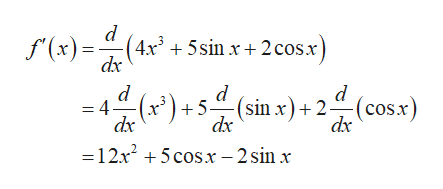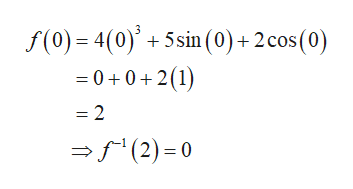# Find (f −1)'(a).f(x) = 4x3 + 5 sin x + 2 cos x,    a = 2 (f −1)'(a) =

Question
712 views

Find (f −1)'(a).

f(x) = 4x3 + 5 sin x + 2 cos x,    a = 2

(f −1)'(a) =

check_circle

Step 1

Consider the function f(x) =4x3+5sinx+2cosx.

Compute the derivative of the given function as followshelp_outlineImage Transcriptionclosed f(x) dr +5sin x+ 2 cosx d d =4- dx (sin x)+2(cosx) dx dx =12x5cosx-2sin x fullscreen
Step 2

By inspection, it is observed that the value of the fu...help_outlineImage Transcriptionclosef(0) 4(0)5 sin (0) + 2 cos (0) -00 2(1) = 2 (2) 0 fullscreen

### Want to see the full answer?

See Solution

#### Want to see this answer and more?

Solutions are written by subject experts who are available 24/7. Questions are typically answered within 1 hour.*

See Solution
*Response times may vary by subject and question.
Tagged in

### Derivative